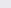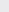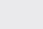Course Content

0/4

0/3

0/1

0/2

0/1

0/4

0/3

0/5

0/3

0/5

0/5

0/1

0/6

0/2

0/4

0/5

16.3 GeometricProgression 等比数列
1.
Each year, the price of an electric equipment increase by 5% of its price in the previous year. Given that the price of the electric instrument is RM800 at the beginning. State its price after n years, in terms of n. Hence, find the price after 10 years, to the nearest ringgit.

RM800(1.05), RM1303
2.Figure beside shows a triangle ABC with right angle at B, angle ACB = β and BC = a. Point D is the leg of the perpendicular line from B to AC, and the same way to define E and F.

(a) State angle ABD in terms of β.

(b) State BD and DE in terms of a and β.

(c) Show that the lengths of CB, BD, DE are in geometric progression.

(a) β

(b) a sinβ, a sinβ

3.
A circle with radius 10cm is divided into 4 sectors where the areas of the sectors are in geometric progression. Given that the area of the largest sector is 8 times of the area of the smallest sector, find the area of the largest sector.cm4.
In an G.P. if the sum of the first six terms is nine time of the sum of first three terms, find the common ratio.

If a, b, c are in G. P., prove thatin A. P.